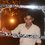# How to do such questions?

I have doubt regarding the solution of the following question,

"Block $A$ of mass $m$ is performing SHM of amplitude $a$. Another block $B$ of mass $m$ is gently placed on A when it passes through mean position and $B$ sticks to $A$. Find amplitude of new SHM."

Given solution,

For mass $m:\frac { 1 }{ 2 } m{ u }^{ 2 }=\frac { 1 }{ 2 } m{ \omega }^{ 2 }{ a }^{ 2 }$ ----$\boxed{1}$

For mass $2m:\frac { 1 }{ 2 } 2m{ v }^{ 2 }=\frac { 1 }{ 2 } 2m\left( \frac { \omega }{ \sqrt { 2 } } \right) ^{ 2 }{ A }^{ 2 }$

By conservation of momentum, $v=\frac { u }{ 2 }$

$\therefore \frac { 1 }{ 2 } 2m{ \left( \frac { u }{ 2 } \right) }^{ 2 }=\frac { 1 }{ 2 } 2m\left( \frac { \omega }{ \sqrt { 2 } } \right) ^{ 2 }{ A }^{ 2 }$ ----$\boxed{2}$

Dividing $\boxed{1}$ by $\boxed{2}$

$4=\frac { 2{ a }^{ 2 } }{ { A }^{ 2 } }$

New amplitude, $A=\frac { a }{ \sqrt { 2 } }$

What I don't get is that how $\frac { \omega }{ \sqrt { 2 } }$ came in step $\boxed{2}$. Could someone please help?Note by Anandhu Raj
5 years, 2 months ago

This discussion board is a place to discuss our Daily Challenges and the math and science related to those challenges. Explanations are more than just a solution — they should explain the steps and thinking strategies that you used to obtain the solution. Comments should further the discussion of math and science.

When posting on Brilliant:

• Use the emojis to react to an explanation, whether you're congratulating a job well done , or just really confused .
• Ask specific questions about the challenge or the steps in somebody's explanation. Well-posed questions can add a lot to the discussion, but posting "I don't understand!" doesn't help anyone.
• Try to contribute something new to the discussion, whether it is an extension, generalization or other idea related to the challenge.
• Stay on topic — we're all here to learn more about math and science, not to hear about your favorite get-rich-quick scheme or current world events.

MarkdownAppears as
*italics* or _italics_ italics
**bold** or __bold__ bold
- bulleted- list
• bulleted
• list
1. numbered2. list
1. numbered
2. list
Note: you must add a full line of space before and after lists for them to show up correctly
paragraph 1paragraph 2

paragraph 1

paragraph 2

[example link](https://brilliant.org)example link
> This is a quote
This is a quote
    # I indented these lines
# 4 spaces, and now they show
# up as a code block.

print "hello world"
# I indented these lines
# 4 spaces, and now they show
# up as a code block.

print "hello world"
MathAppears as
Remember to wrap math in $$ ... $$ or $ ... $ to ensure proper formatting.
2 \times 3 $2 \times 3$
2^{34} $2^{34}$
a_{i-1} $a_{i-1}$
\frac{2}{3} $\frac{2}{3}$
\sqrt{2} $\sqrt{2}$
\sum_{i=1}^3 $\sum_{i=1}^3$
\sin \theta $\sin \theta$
\boxed{123} $\boxed{123}$

## Comments

Sort by:

Top Newest

$\omega = \sqrt{\dfrac{k}{m}}$ where $F = - kx = ma$ is the restoring force.

$k$ is constant, as the restoring force is assumed to not change.

- 5 years, 2 months ago

Log in to reply

Thank you very much, Sir! Do you have a problem if I mention your name in this question?

- 5 years, 2 months ago

Log in to reply

Absolutely not, thank you for the honour!

- 5 years, 2 months ago

Log in to reply

Also, I think you may have accidentally posted the same note many times, just delete the other ones.

- 5 years, 2 months ago

Log in to reply

×

Problem Loading...

Note Loading...

Set Loading...